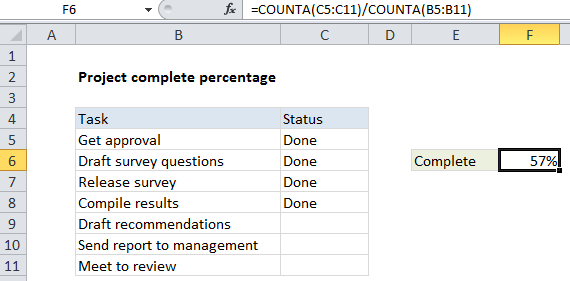## Excel Office

Excel How Tos, Tutorials, Tips & Tricks, Shortcuts

# How to calculate project complete percentage in Excel

To calculate the percentage complete for a project with a list of tasks, you can use a simple formula based on the COUNTA function.

## Formula

`=COUNTA(range1)/COUNTA(range2)`## Explanation

In the example shown, the formula in F6 is:

`=COUNTA(C5:C11)/COUNTA(B5:B11)`

### How this formula works

At the core, this formula simply divides tasks complete by the total task count:

`=complete/total`

which is then formatted as a percentage.

Also See:   How To Use AGGREGATE function to sum a range with errors in Excel

To count complete tasks, we count non-blank cells in the range C5:C11:

`=COUNTA(C5:C11)`

To count total tasks, we count non-blank cells in the range C5:C11:

`COUNTA(B5:B11)`

Unlike the COUNT function, which counts only numeric values, COUNTA will count cells that include numbers or text.

1.